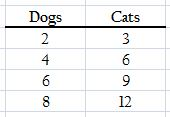# Solving Ratio Problems With Tables, Tape Diagrams & Double Number Lines

Instructions:

Choose an answer and hit 'next'. You will receive your score and answers at the end.

question 1 of 3

### What is a ratio problem?

Create Your Account To Take This Quiz

As a member, you'll also get unlimited access to over 75,000 lessons in math, English, science, history, and more. Plus, get practice tests, quizzes, and personalized coaching to help you succeed.

Try it risk-free for 30 days. Cancel anytime.

### 2. Joe tells you that the ratio of dogs to cats in one area is 2:3. If there are four dogs, how many cats will there be? Use the table to find the solution.Create your account to access this entire worksheet
A Premium account gives you access to all lesson, practice exams, quizzes & worksheets
Quizzes, practice exams & worksheets
Certificate of Completion
Create an account to get started

These study tools will quiz you on several methods for solving ratio problems, including table, tape diagram, and double number line. Quiz questions will ask you to describe a ratio problem and find values to complete ratios in practice problems

## Quiz & Worksheet Goals

In these assessments you'll be tested over your ability to:

• Define ratio problem
• Identify relationships between values
• Find the values of ratios
• Solve for missing values in ratios in practice problems

## Skills practiced

You can practice the following skills in this worksheet and quiz combination:

• Reading comprehension - ensure that you draw the most important information from the related mathematics lesson
• Problem solving - use acquired knowledge to solve ratio practice problems
• Knowledge application - use your knowledge to answer questions about the different methods to find missing values in ratios

You can learn more about methods for solving ratio problems in the accompanying lesson Solving Ratio Problems With Tables, Tape Diagrams & Double Number Lines. This covers the following objectives:

• Recall what a ratio is
• Solve example ratios with several methods, including tables, tape diagrams, and double number lines
• Define each method of solving ratios, terms include table, tape diagram, and double number line
Final ExamCommon Core Math Grade 6 - Ratios & Proportional Relationships: Standards
Status: Not Started
Chapter ExamSolving Ratio & Unit Rate Problems: CCSS.Math.Content.6.RP.A.3A-B
Status: Not Started

Support